# Method of characteristics

(diff) ← Older revision | Latest revision (diff) | Newer revision → (diff)

A method for the numerical integration of equations of hyperbolic type. In the domain of hyperbolicity there is a linear combination of initial equations in which there occur only interior derivatives along characteristic surfaces. Then the equations to be solved simplify substantially. In the method of characteristics the solution is computed on a characteristic grid, which is constructed in the process of calculation, and so the domain of dependency of the solution can be determined exactly. For the method of characteristics the existence of a solution and its convergence have been proved. The widest application of the method of characteristics is in the solution of problems of the mechanics of continuous media (see ). For example, the equations in characteristic form(1)(2)

are a linear combination of the traditional equations of gas dynamics: continuity, momentum and energy. Here and belowis the density,is the velocity,is the intrinsic energy of a unit mass,is the pressure,is the temperature,is the spatial coordinate, andis the time. The Cauchy problem asks for a solution in the domain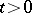for given data on the line. Entropy is the name for an integral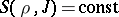of the equation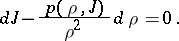Then (1) has the form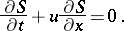(1prm)

On the left-hand sides of (1prm) and (2) there stand the derivatives,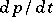,, taken in the directions(3)

and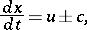(4)

the so-called characteristics. The system (1), (2) has three families of real characteristics. Along the characteristics (3) the relationholds, and along the characteristic (4) the relations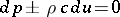(5)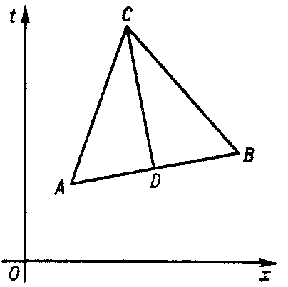Figure: m063580a

hold. Through the point(see Fig.) there passes a characteristic (4) on the side of increasing,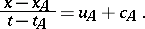Through a point close toon the right, say, there passes a characteristic (4) of another familywhereis the point of intersection of the characteristics. When one replaces the differential relations (5), which hold along characteristics, by differences, one obtains the algebraic systemfrom which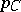and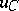can be determined. Fromone draws the characteristic (3),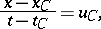up to the intersection withat. The value of the entropyatis determined by means of interpolation between the pointsand(here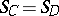). From the equations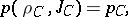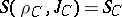one can find the values of the intrinsic energy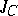and the density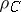at. When the data at the two pointsandare known, one can find the solution atfor large values of. This procedure of computations is repeated for every pair of points. Then, by using new pointsinstead of the originaland, one takes the next step in. The calculation is made for the required values of. However, since the equations of gas dynamics are non-linear, the calculation may come to a stop at a certain moment of time if the characteristics of one family touch each other or intersect.

The difference scheme thus described is of the first order of accuracy (an analogue of Euler's method of polygonal lines for solving ordinary differential equations). An increase in accuracy can be achieved by repetitions of the calculations, etc.

Using the method of characteristics one can solve stationary multi-dimensional problems in a domain of hyperbolicity (for gas dynamics — of supersonic flow). One can also determine the position of secondary shock waves at places where the characteristics of a single family intersect or touch. By the method of characteristics one can tackle only problems with a small number of discontinuities, since under an accumulation of singularities the computations become tedious. A computation by the method of characteristics consists of a number of elementary problems: the calculation of an interior point, of a point on the shock wave, or on a body around which the flow is taking place, etc.

One can construct numerical schemes for the method of characteristics, which make it possible to carry out the calculations in "layers" , the grid-characteristic method (see ).

How to Cite This Entry:
Method of characteristics. Encyclopedia of Mathematics. URL: http://encyclopediaofmath.org/index.php?title=Method_of_characteristics&oldid=15839
This article was adapted from an original article by Yu.M. Davydov (originator), which appeared in Encyclopedia of Mathematics - ISBN 1402006098. See original article